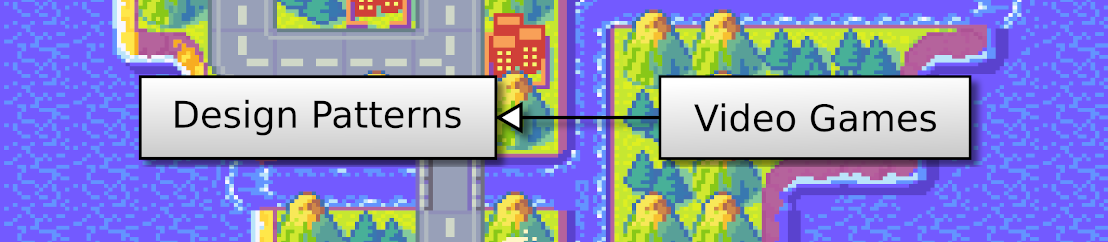## Discover Python and Patterns (14): Collisions

In this post, I add enemies and I show you how to test if the tank collides one of them.

This post is part of the Discover Python and Patterns series

## Objective

I propose to add two towers at locations (10,3) and (10,5). I use two sprites to draw them, one for the base, and the other for the gun:

Locations are cell coordinates, from left to right (x coordinate) and from top to bottom (y coordinate). The lower coordinate value is 0, and the highest one is the width or height of the world minus one. So, with a world of 16 per 10 cells, the x coordinate goes from 0 to 15, and the y coordinate goes from 0 to 9:

Looking at this screenshot, you can say that the tank is at the location (5,4).

## Program structure

I still use the two classes from the previous post:

I created two new attributes in the `GameState` class:

• New attribute `tower1Pos`: the location of the first tower
• New attribute `tower2Pos`: the location of the second tower

## Add towers to the world

As in the previous posts, I put all the data related to the world into an instance of the `GameState`. I initialize this data in the constructor the `GameState` class:

``````def __init__(self):
self.worldSize = Vector2(16,10)
self.tankPos = Vector2(5,4)
self.tower1Pos = Vector2(10,3)
self.tower2Pos = Vector2(10,5)      ``````

## Draw a tower

The drawing of a tower is similar to the one we did for the tank:

``````spritePoint = self.gameState.tower1Pos.elementwise()*self.cellSize
texturePoint = Vector2(0,1).elementwise()*self.cellSize
textureRect = Rect(int(texturePoint.x), int(texturePoint.y), int(self.cellSize.x),int(self.cellSize.y))
self.window.blit(self.unitsTexture,spritePoint,textureRect)
texturePoint = Vector2(0,6).elementwise()*self.cellSize
textureRect = Rect(int(texturePoint.x), int(texturePoint.y), int(self.cellSize.x),int(self.cellSize.y))
self.window.blit(self.unitsTexture,spritePoint,textureRect)``````

Let's take this process line by line. The first line creates a variable `spritePoint` that contains the pixel location on the screen, where the sprite appears:

``spritePoint = self.gameState.tower1Pos.elementwise()*self.cellSize``

The two variables in the multiplication are Pygame `Vector2` instances: it is like tuples of two floats (x,y), except that there are many nice features to ease computations. For instance, if you use the `elementwise()` method like in the line above, it allows you to do an element-wise multiplication:

``````spritePoint = Vector2()
spritePoint.x = self.gameState.tankPos.x * self.cellSize.x
spritePoint.y = self.gameState.tankPos.y * self.cellSize.y``````

As a result, it reduces three lines of code into one.

The second line of the drawing of a tower computes the pixel location of the tower sprite in the image tileset:

``texturePoint = Vector2(0,1).elementwise()*self.cellSize``

This sprite is in the first column of the second row of the tile, so cell coordinates (0,1). If the pixel size of cells is (64,64), then the pixel location of this sprite if (0,64).

The third line creates a Pygame `Rect` instance with the rectangle that contains the tower sprite in the tileset:

``textureRect = Rect(int(texturePoint.x), int(texturePoint.y), int(self.cellSize.x),int(self.cellSize.y))``

We must convert float values into integer values: to do so, you can use the `int` type as if it was a function. Then, `int(x)` is the cast of `x` into an `int`.

The fourth line draws the sprite on screen:

``self.window.blit(self.unitsTexture,spritePoint,textureRect)``

The `blit()` method from the Pygame `Surface` class (what is `self.window`) needs three arguments: the tileset (here `self.unitsTexture`), the pixel location on the screen (here `spritePoint`), and the rectangle that contains the sprite in the tileset (here `textureRect`).

The last lines are very similar to the first ones, except that we don't recompute `spritePoint`: the aim is to draw a gun at the same location. These lines are a copy of lines 2-4, with a change for the location of the sprite: it is a gun sprite at (0,6) instead of a tower base sprite at (0,1).

## Collisions

If you add the previous changes in the program, then you will see the two towers on the screen. However, if you move the tank, it can go over the towers.

In the previous program, we were already testing tank locations. More specifically, we forbid it to go outside the world:

``````def update(self,moveTankCommand):
self.tankPos += moveTankCommand

if self.tankPos.x < 0:
self.tankPos.x = 0
elif self.tankPos.x >= self.worldSize.x:
self.tankPos.x = self.worldSize.x - 1

if self.tankPos.y < 0:
self.tankPos.y = 0
elif self.tankPos.y >= self.worldSize.y:
self.tankPos.y = self.worldSize.y - 1``````

With this approach, the tank location is first updated (line 2) and then corrected if wrong (lines 4-11). It works fine in the case where the world is empty. If it is not the case, we can't be sure that the corrected location is empty!

Another approach is first to compute the targetted location, and then update the true location if it is correct:

``````def update(self,moveTankCommand):
newTankPos = self.tankPos + moveTankCommand

if  newTankPos.x >= 0 and newTankPos.x < self.worldSize.x \
and newTankPos.y >= 0 and newTankPos.y < self.worldSize.y \
and newTankPos != self.tower1Pos and newTankPos != self.tower2Pos:
self.tankPos = newTankPos``````

The second line stores the target location in `newTankPos`. Then, all the remaining lines is a long `if`statement that does all the checks. Note that there is a backslash `\` at the end of lines 4 and 5: this is to split the long statement into multiple lines to make it more readable. You can remove them and write all the `if` condition on a single line if these backslashes are not clear to you. The conditions to meet are:

• To be inside the world: tank (x,y) location must be between (0,0) and (world width-1,world height-1). Remind that coordinates are always between zero and the size minus one.
• Not to be at the location of tower 1. The operator `!=` means "is different from". So, `newTankPos != self.tower1Pos` means "the new tank location is different from the location of the first tower`.
• Not to be at the location of tower 2.